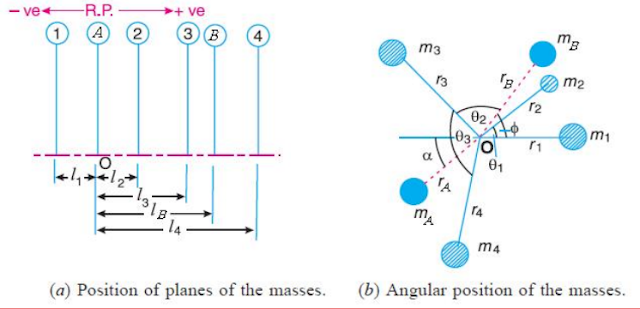## Tuesday, 8 October 2019

### Balancing of Several Masses Rotating in Different Planes

When several masses revolve in different planes, they can be transferred to a 'Reference plane' (R.P), which may be defined as the plane passing through a point on the axis of rotation and perpendicular to it. The effect of transferring a revolving mass (in one plane) to a reference plane is to cause a force of magnitude equals to the centrifugal force of the revolving mass to act in the reference plane, together with a couple of magnitude equal to the product of the force and the distance between the plane of rotation and the reference plane. In order to have a complete balance of the several revolving masses in different planes, the following conditions must be satisfied:
1. The resultant force must be zero (i.e All the forces in the reference plane must be balanced)
2. The resultant couple must be zero (i.e The couple about the reference plane must be balanced)
Let us now consider four masses revolving in different planes 1, 2, 3 and 4 respectively as shown in Fig., (a), and their relative angular positions are shown in Fig (b).,The magnitude of the balancing masses mand min planes A and B may be obtained as discussed below.
1. Take one of the plane say A, as reference plane (R.P).
2. The distances of the other planes to the left of the reference plane is taken as negative, and those are present on the right as Positive.
3. Tabulate the planes data in the same order from left to right as shown in below table.

4. A couple may be represented by a vector drawn perpendicular to the plane of couple. Couple C1 is obtained by transferring mto the reference plane through O. The couple obtained is m1 . r1 . land it acts in a plane through and perpendicular to the paper. The vector representing this couple is drawn in the plane of the paper and perpendicular to Omas OCshown by in Fig., Similarly the remaining vectors for remaining masses is calculated and shown in Fig.,
5. The couple vectors as discussed above, are turned counter clockwise through a right angle for convenience of drawing without changing relative positions.
6. Now draw the couple polygon as shown in Fig., The couples about the reference plane must be balance. i.e., the resultant couple must be zero.

7. Now draw the force polygon as shown in Fig., The forces in the reference plane must balance. i.e., the resultant forces must be zero.

From the above expression, the value of balancing mass min the plane 'A' may be obtained and the angle of inclination of this mass with the horizontal may be measured from Fig., (Angular positions of masses